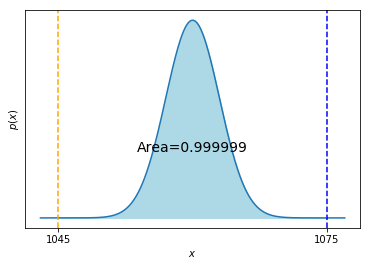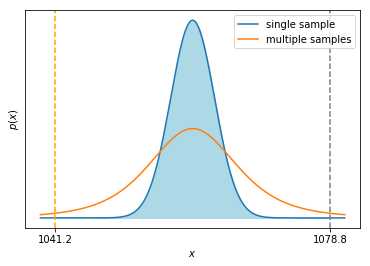# Hypothesis Tests (Parametric)¶

## Unit 10, Lecture 1¶

Numerical Methods and Statistics

Langley: Pages 137-189, 199-211, 230-245

## Goals:¶

1. Understand the meaning of a null hypothesis
2. Be able to construct a null hypothesis
3. Be able to calculate a p-value, understand its meaning, and test it against significance level
4. Visualize the intervals used to compute p-values for $z$ and $t$ tests
In :
import numpy as np
import matplotlib.pyplot as plt
from math import sqrt, pi, erf
import scipy.optimize


# Hypothesis Testing¶

Hypothesis: Going to college increases your starting salary. How do you prove this?

It's not really possible to prove this directly. We can, however, disprove the opposite hypothesis. We construct the opposite hypothesis to what we're interested in, called the null hypothesis. The null hypothesis is an assumption of no-difference and/or no-correlation.

In our example, this seems simple at first: Going to college has no effect on your salary.

But, maybe the null hypothesis is: For People who qualified and were accepted to college, attending college has no effect on their salary.

Or it might be People who can afford, are smart enough, and motivated enough to go to college but did not, have the same salary as those that did.

Let's assume we know well enough what our null hypothesis is. Hypothesis testing is the ability to use statistics to disprove the null hypothesis

Null Hypothesis: The simplest explanation, typically meaning no correlation or that all data is from the same population. Because a simpler explanation is preferred to a more complex one, the null hypothesis should be our default belief. We construct our null hypothesis as sort-of the opposite of what we want to study. For example, if we want to know if a sample is significantly different than our population, our null hypothesis is that it is not significantly different and then we aim to disprove the null hypothesis. Hypothesis testing is about showing significance by disproving or rejecting the null hypothesis.

## Hypothesis Testing¶

We construct a null hypothesis and take it to be true. For example, we believe college has no influence on income. This allows us to build a simple probability model. For colelge then, we might take income to be normally distributed. Then we see how likely our observed data is according to that null hypothesis model. For example, we check to see if the sample mean of people who graduated from college matches our null hypothesis mean.

## Hypothesis Test Example¶

I open up a cash4gold store and people bring me their jewlery. I know the probability distribution for gold melting is normal with mean 1060 $^{\circ}$C and my measurements have a standard deviation of 3$^{\circ}$C. I melt a sample at 1045 $^{\circ}$ C and want to know if I should be suspicious.

Null Hypothesis: The sample is gold

Let's see if I can disporve this. If the sample is gold, what is the probability of that measurement? Zero, because it's a single point. Let's ask though how big of an interval would we need to include that measurement.

In :
from scipy import stats as ss

Z = abs(1045 - 1060.) / 3
interval_p = ss.norm.cdf(Z) - ss.norm.cdf(-Z)

print(interval_p)

0.999999426697

In :
import numpy as np
import matplotlib.pyplot as plt

sample = 1045
mu = 1060
w = mu - sample
x = np.linspace(mu - w  - 2, w + mu + 2, 1000)
y = ss.norm.pdf(x, loc=mu, scale=3)
plt.plot(x,y)
plt.fill_between(x, y, where= np.abs(x - mu) < w, color='lightblue')
plt.text(mu,np.max(y) / 3, 'Area={}'.format(np.round(interval_p,6)), fontdict={'size':14}, horizontalalignment='center')
plt.axvline(mu - w, linestyle='--', color='orange')
plt.axvline(mu + w, linestyle='--', color='blue')
plt.xticks([mu - w, mu + w], [sample, mu + w])
plt.yticks([])
plt.ylabel(r'$p(x)$')
plt.xlabel(r'$x$')
plt.show()We would expect to see values outside or at the boundary of this interval 0.00000001% of the time.

## What is signficiant?¶

Would we call it significant if it was 0.1%? what about 1%? It turns out the convention is 5%. This is called our $\alpha$ or significance level.

We saw in the cash4gold example how to compare a single sample with a known population. What about when we don't know the standard deviation of the population?

I open up a cash4gold store and people bring me their jewlery. I know the probability distribution for gold melting is normal with mean 1060 $^{\circ}$C. I do not know the standard deviation. If I get a sample that melts at 1045$^\circ{}$C, should I be suspicious?

I don't know. We have no way of estimating the standard deviation with one sample, so we can't say anything. If we have at least 3 samples though, we can compute the sample standard deviation

This leads us to the beautiful part about hypothesis testing: since we're assuming the null hypothesis, that the samples are gold, we can use what we learned about normal distributions to estimate the population standard deviation from our samples.

## General Strategy¶

The general approach for hypothesis tests is as follows:

1. Start with a null hypothesis, $H_0$, that corresponds to a probability distribution/mass function
2. Compute the probability of an interval which inculdes values as extreme as your sample data. Note this may be single- or double-sided depending on if "extreme" means both above and below or only above/below the mean. This is your $p$-value.
3. Reject the null hypothesis if the $p$-value is below your significance level ($\alpha$), which is generally 0.05

# Student's $t$-Test¶

I open up a cash4gold store and people bring me their jewlery. I know the probability distribution for gold melting is normal with mean 1060 $^{\circ}$C. I do not know the standard deviation. Someone brings in 6 samples and they melt at 1035, 1050, 1020, 1055, and 1046 $^{\circ}$C. Should I reject the null hpyothesis, that these are gold?

#### Logical Steps:¶

1. Assuming the null hypothesis, compute our uncertainty in the true mean confidence interval. This is our probability distribution
2. We happen to have the true mean, so then we see how big the confidence interval has to be to include it. This is us constructing our probability interval
3. Take the area of that interval, our $p$-value, and compare with the significance level
In :
import numpy as np
import matplotlib.pyplot as plt

samples = np.array([1035., 1050., 1020., 1055., 1046.])
sigma = np.sqrt(np.var(samples, ddof=1))
sample_mean = np.mean(samples)
mu = 1060
w = mu - sample_mean

x = np.linspace(mu - w  - 2, w + mu + 2, 1000)
y1 = ss.norm.pdf(x, loc=mu, scale=3)
y2 = ss.t.pdf(x, loc=mu, scale=sigma / np.sqrt(len(samples)), df=len(samples) - 1)
plt.plot(x,y1, label='single sample')
plt.plot(x,y2, label='multiple samples')
plt.fill_between(x, y1, where= np.abs(x - mu) < w, color='lightblue')

plt.axvline(mu - w, linestyle='--', color='orange')
plt.axvline(mu + w, linestyle='--', color='gray')
plt.xticks([mu - w, mu + w], [sample_mean, mu + w])
plt.yticks([])
plt.ylabel(r'$p(x)$')
plt.xlabel(r'$x$')
plt.legend(loc='best')
plt.show()In :
samples = np.array([1035., 1050., 1020., 1055., 1046.])
sigma = np.sqrt(np.var(samples, ddof=1))
sample_mean = np.mean(samples)
true_mean = 1060.

print(sigma, sample_mean, true_mean)

T = (sample_mean - true_mean) / (sigma / np.sqrt(len(samples)))
print(T)

13.953494186 1041.2 1060.0
-3.01272766638


Now we have a $T$ and we know $P(T)$. However, just like the $zM$ test, we can't compute $P(T)$ since that's a single point and we're using a continuous distribution. So instead, we build an interval and see how big it must be to catch that $T$.

Specifically, we want to find $\int_{-T}^T p(t)\,dt$

In :
print('T = ', T)

p = ss.t.cdf(T, len(samples) - 1) # The integral from -infinity to T
print(p, 'Is the single sided p-value')

p = 1 - (ss.t.cdf(-T, len(samples) - 1) - ss.t.cdf(T, len(samples) - 1)) # 1- The integral from -T to T
print(p, 'Is the double sided p-value')

print('notice, just using 2 * the single-sided value gives the same answer')

T =  -3.01272766638
0.0197221623052 Is the single sided p-value
0.0394443246103 Is the double sided p-value
notice, just using 2 * the single-sided value gives the same answer


## What if accidentally reverse our T-value?¶

In :
T = (true_mean - sample_mean) / (sigma / sqrt(len(samples)))
print (T)

p = (scipy.stats.t.cdf(T, len(samples) - 1))
print ('CDF gives: ', p)
print ('Recognize that includes from -infty up to a positive T, so we need to find the complementary area')

print (1 - p, 'Is the single sided p-value')
print ((1 - p) * 2, 'Is the double sided p-value')

3.01272766638
CDF gives:  0.980277837695
Recognize that includes from -infty up to a positive T, so we need to find the complementary area
0.0197221623052 Is the single sided p-value
0.0394443246103 Is the double sided p-value


# $zM$ Test¶

Data Type: Measurements and Ranks

Compares: A single sample vs a normally distributed population

Null Hypothesis: The sample came from the population

Conditions: Standard deviation of population is known

Related Test 1: Student's $t$-test, which is used when the standard deviation is not known

Python: Integrate an interval with a Z-statistic and erf or scipt.stats.norm.cdf

# Student's $t$-test¶

Data Type: Measurements and Ranks

Compares: A set of samples vs a normally distributed population

Null Hypothesis: The sample came from the population

Conditions: Standard deviation of population is not known

Related Test 1: $zM$ test, which is used when standard deviation is known

Python: Integrate an interval with a T-stastic and scipt.stats.t.cdf